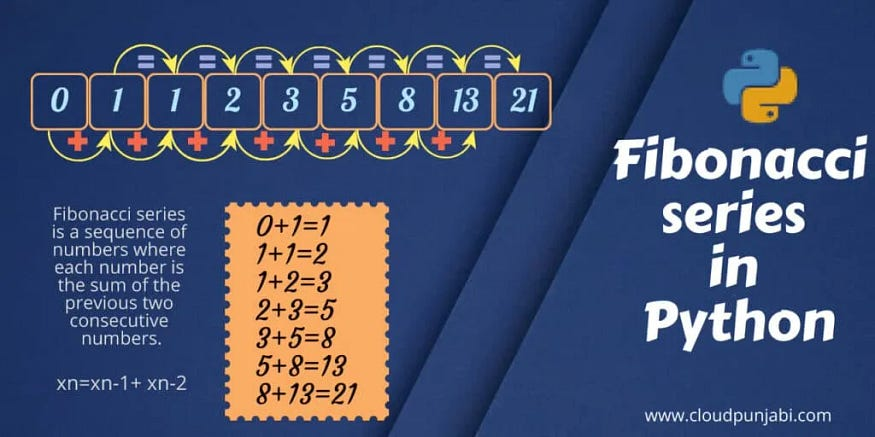# Fibonacci Series in Python | 5 Best Programs

## 5 Python programs to print the Fibonacci series.

Do you have questions related to the Fibonacci series in Python? Like, what is the Fibonacci series, and how to write Python code for the Fibonacci series? You will get answers to all these questions in this article. We will also discuss different ways of writing the Python program for the Fibonacci series.### What is the Fibonacci series in Python?

Fibonacci series is a sequence of numbers where each number is the sum of the previous two consecutive numbers. The series begins with 0 and 1.

### The formula of the Fibonacci series

xn=xn-1+ xn-2 (where 'n' is the term number)

### The logic of the Fibonacci series

• First term: 0
• Second term: 1
• Third term: (0+1)= 0
• Fourth term: (1+1)=2
• Fifth term: (2+1)=3

### Ways to write Fibonacci series in Python

There are different ways to write a python program for the Fibonacci series. Each program is different works on different algorithms. We will discuss each method to print the Fibonacci series in python with their algorithm and example, which software development companies commonly employ.

### 1. Fibonacci series in Python without recursion

This is the most basic python program to print the Fibonacci series. And In fact, this is the also most efficient Fibonacci program. Let’s understand this algorithm more deeply.

#### Step-by-step algorithm of Fibonacci program

1. Initialize the first and second variables 0 and 1 respectively.
2. Print the first and the second variable.
3. Now using for loop up to the given number. for instance 18
4. Now under for loop, initialize the third variable equal to the sum of the first and second variable.
5. Print third.
6. Now, store value second in first and then, store the value of third in second.
7. Further, from step 4 process is repeated for the given number of terms i.e., 18.
8. Stop

#### Python code

Let’s see the Python code and find out what will be the output of the following Python code:

``````#python program for fibonacci series without recursion
first = 0
second = 1
print(first)
print(second)
for x in range(1,9):
third = first + second
print(third)
first,second=second,third
``````

#### Output:

This is the output for the Fibonacci series to 10 terms.

``````#Output
0
1
1
2
3
5
8
13
21
34
``````

### 2. Fibonacci program using for loop

In this Fibonacci code, we will use for loop with range() to specify the number of terms of the Fibonacci series.

#### Python code

Let’s see the code of the Fibonacci series in python using for loop

``````#python program for fibonacci series using for loop
n=int(input("Enter the number of terms: "))
a=0
b=1
if n<=0:
print("The Output of your input is",a)
else:
print(a,b,end=" ")
for x in range(2,n):
c=a+b
print(c,end=" ")
a=b
b=c
``````

#### Output

Input= 8

``````Enter the number of terms: 8
0  1  1  2  3  5  8  13
``````

### 3. Fibonacci program using while loop

This is the most used Fibonacci series program in Python. In this Python code, we will use a while loop to specify the number of terms of the Fibonacci series.

#### Python code

Let’s see the code using for loop

``````#Python program to generate Fibonacci series until nth term
n = int(input("Enter the value of nth term: "))
a = 0
b = 1
c = 0
count = 1
print("Fibonacci Series: ", end = " ")
while(count <= n):
print(c, end = " ")
count += 1
a = b
b = sum
sum = a + b
``````

#### Output

Input = 10

``````Enter the value of nth term: 10
Fibonacci Series: 0  1  1  2  3  5  8  13  21  34
``````

### 4. Fibonacci program using recursion

Recursion is a function calling itself again and again. This method is very powerful in Python programming. So, Let’s write the recursive Python code for the Fibonacci series using the Python define function which is an inbuilt function in Python.

#### Recursive code for fibonacci series

``````#Recursive function to print Fibonacci series
def rec_fibo(n):
if n <=1:
return n
else:
return(rec_fibo(n-1) + rec_fibo(n-2))nterms=int(input("How many terms of series are required? "))
print("Fibonacci series is generated below : ")
for i in range(nterms):
print(rec_fibo(i))
``````

#### Output

Input =9

``````How many terms of series are required? 9
Fibonacci series is generated below :
0
1
1
2
3
5
8
13
21
``````

### Conclusion

In conclusion, We have discussed 5 programs for the Fibonacci series in Python. Now, you know each program with its algorithm and example. I found the recursive program best. Hope that now you know all about Fibonacci in Python.